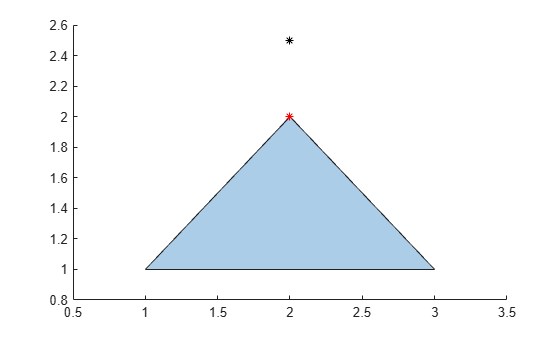# nearestvertex

Query nearest `polyshape` vertex

## Syntax

``[vertexid,boundaryid,ind] = nearestvertex(polyin,x,y)``
``[vertexid,boundaryid,ind] = nearestvertex(polyin,P)``

## Description

example

````[vertexid,boundaryid,ind] = nearestvertex(polyin,x,y)` finds the vertex of a scalar `polyshape` object that is nearest to each 2-D query point. The coordinates of the query points are specified in `x` and `y`. The output contains three vectors: `vertexid` contains the row number in `polyin.Vertices` corresponding to the nearest vertex for each query point.`boundaryid` contains the boundary index of `polyin` corresponding to the nearest vertex for each query point.`ind` contains the nearest vertex index in the boundary `boundaryid` for each query point. ```
````[vertexid,boundaryid,ind] = nearestvertex(polyin,P)` specifies the query points as a 2-column matrix `P` whose first column contains the x-coordinates and whose second column contains the corresponding y-coordinates.```

## Examples

collapse all

Create a `polyshape` object and compute the nearest vertex of the `polyshape` to the query point (2,1.8).

```P = [1 1; 3 1; 2 2]; polyin = polyshape(P); [vertexid,boundaryid,ind] = nearestvertex(polyin,2,1.8);```

Plot the `polyshape` and the query point in black.

```plot(polyin) hold on plot(2,2.5,'k*')```

Now plot the nearest vertex in red. The `vertexid` value enables you to index into the row of `polyin.Vertices` corresponding to the nearest vertex, accessing its coordinates.

```plot(polyin.Vertices(vertexid,1),polyin.Vertices(vertexid,2),'r*') hold off```## Input Arguments

collapse all

Input `polyshape`, specified as a scalar `polyshape` object.

Input coordinates of 2-D query points, specified as scalars or vectors. `x` and `y` must have the same length.

Data Types: `double` | `single` | `int8` | `int16` | `int32` | `int64` | `uint8` | `uint16` | `uint32` | `uint64`

Input vertices of 2-D query points, specified as a 2-column matrix. The first column of `P` contains the x-coordinates and the second column contains the corresponding y-coordinates.

Data Types: `double` | `single` | `int8` | `int16` | `int32` | `int64` | `uint8` | `uint16` | `uint32` | `uint64`

## Output Arguments

collapse all

Vertex ID, returned as a scalar or vector. `vertexid` contains the row number in the input `polyshape` corresponding to the nearest vertex for each query point. `vertexid` is the same length as the query point vectors or array.

Data Types: `double`

Boundary ID, returned as a scalar or vector. `boundaryid` contains the boundary index of the input `polyshape` corresponding to the nearest vertex for each query point. `boundaryid` is the same length as the query point vectors or array.

Data Types: `double`

Index, returned as a scalar or vector. `ind` contains the nearest vertex index in the boundary `boundaryid` for each query point.

Data Types: `double`